The Australian Journal of Mathematical Analysis and Applications

 Home News Editors Volumes RGMIA Subscriptions Authors Contact

ISSN 1449-5910

Sorry death is imminent for file: ../public_html/searchroot/files/tex/v19n2/v19i2p4.tex
You searched for jah
Total of 68 results found in site

10: Paper Source PDF document

Paper's Title:

Topological Aspects of Scalarization in Vector Optimization Problems.

Author(s):

Peter I. Kogut, Rosanna Manzo and Igor V. Nechay

Department of Differential Equations,
Dnipropetrovsk National University, Naukova STR.,
13, 49010 Dnipropetrovsk,
Ukraine

p.kogut@i.ua

Universitŕ di Salerno,
Dipartimento di Ingegneria dell'Informazione e Matematica Applicata,
Via Ponte don Melillo, 84084 Fisciano (SA),
Italy

manzo@diima.unisa.it

Department of Technical Cybernetics,
Dnipropetrovsk Technical University,
Acad. Lazarjan STR., 2, 49010 Dnipropetrovsk,
Ukraine

i.nechay@i.ua

Abstract:

In this paper, we study vector optimization problems in partially ordered Banach spaces. We suppose that the objective mapping possesses a weakened property of lower semicontinuity and make no assumptions on the interior of the ordering cone. We derive sufficient conditions for existence of efficient solutions of the above problems and discuss the role of topological properties of the objective space. We discuss the scalarization of vector optimization problems when the objective functions are vector-valued mappings with a weakened property of lower semicontinuity. We also prove the existence of the so-called generalized efficient solutions via the scalarization process. All principal notions and assertions are illustrated by numerous examples.

8: Paper Source PDF document

Paper's Title:

Coefficient Estimates for Certain Subclasses of Bi-univalent Sakaguchi Type Functions by using Faber Polynomial

Author(s):

P. Murugabharathi, B. Srutha Keerthi

Mathematics Division,
Chennai - 600 127, India.
E-mail: bharathi.muhi@gmail.com
E-mail: sruthilaya06@yahoo.co.in

Abstract:

In this work, considering a general subclass of bi-univalent Sakaguchi type functions, we determine estimates for the general Taylor-Maclaurin coefficients of the functions in these classes. For this purpose, we use the Faber polynomial expansions. In certain cases, our estimates improve some of those existing coefficient bounds.

7: Paper Source PDF document

Paper's Title:

Generalized Efficient Solutions to One Class of Vector Optimization Problems in Banach Space

Author(s):

Peter I. Kogut, Rosanna Manzo, and Igor V. Nechay

Department of Differential Equations,
Dnipropetrovsk National University,
Naukova str., 13, 49050 Dnipropetrovsk,
Ukraine
p.kogut@i.ua

Dipartimento di Ingegneria Dell’informazione e Matematica Applicata,
Universitŕ di Salerno,
Via Ponte Don Melillo, 84084 Fisciano (Sa),
Italy
manzo@diima.unisa.it

Department of Technical Cybernetics,
Dnipropetrovsk Technical University,
Dnipro
petrovsk,
Ukraine
i.nechay@i.ua

Abstract:

In this paper, we study vector optimization problems in Banach spaces for essentially nonlinear operator equations with additional control and state constraints. We assume that an objective mapping possesses a weakened property of lower semicontinuity and make no assumptions on the interior of the ordering cone. Using the penalization approach we derive both sufficient and necessary conditions for the existence of efficient solutions of the above problems. We also prove the existence of the so-called generalized efficient solutions via the scalarization of some penalized vector optimization problem.

6: Paper Source PDF document

Paper's Title:

On Certain Classes of Harmonic Univalent Functions Based on Salagean Operator

Author(s):

G. Murugusundaramoorthy, Thomas Rosy, and B. A. Stephen

Department of Applied Mathematics and Informatics,
Department of Mathematics, Vellore Institute of Technology,
Deemed University, Vellore - 632014, India.
gmsmoorthy@yahoo.com

Department of Applied Mathematics and Informatics,
Department of Mathematics, Madras Christian College,
Chennai - 600059, India.
drthomasrosy@rediffmail.com

Abstract:

We define and investigate a class of complex-valued harmonic univalent functions of the form f = h + g using Salagean operator where h and g are analytic in the unit disc U = { z : |z| < 1 }. A necessary and sufficient coefficient conditions are given for functions in these classes. Furthermore, distortion theorems, inclusion relations, extreme points, convolution conditions and convex combinations for this family of harmonic functions are obtained.

6: Paper Source PDF document

Paper's Title:

Operators On Frames

Author(s):

Department of Mathematics,
Jahrom University, P.B. 7413188941,
Jahrom,
Iran.

Department of Mathematics,
Jahrom University, P.B. 7413188941,
Jahrom,
Iran.
E-mail: ghorbani@jahromu.ac.ir

Abstract:

In this paper, we first show the conditions under which an operator on a Hilbert space H can be represented as sum of two unitary operators. Then, it is concluded that a Riesz basis for a Hilbert space H can be written as a sum of two orthonormal bases. Finally, the study proves that if A is a normal maximal partial isometry on a Hilbert space H and if {ek}k=1 is an orthonormal basis for H, then {Aek}k=1 is a 1-tight frame for H.

5: Paper Source PDF document

Paper's Title:

On a Class of Uniformly Convex Functions Defined by Convolution with Fixed Coefficient

Author(s):

T. N. Shanmugam, S. Sivasubramanian, and G. Murugusundaramoorthy

Department of Mathematics,
College of Engineering,
Anna University,
Chennai - 600 025,
India.

drtns2001@yahoo.com

Department of Mathematics,
University College of Engineering,
Tindivanam
Anna University-Chennai,
Saram-604 703,
India.
sivasaisastha@rediffmail.com

School of Sciences
and Humanities,
VIT University, Vellore-632 014,
India.
gmsmoorthy@yahoo.com

Abstract:

We define a new subclass of uniformly convex functions with negative and fixed second coefficients defined by convolution. The main object of this paper is to obtain coefficient estimates distortion bounds, closure theorems and extreme points for functions belong to this new class . The results are generalized to families with fixed finitely many coefficients.

4: Paper Source PDF document

Paper's Title:

ψ(m,q)-Isometric Mappings on Metric Spaces

Author(s):

Sid Ahmed Ould Beinane, Sidi Hamidou Jah and Sid Ahmed Ould Ahmed Mahmoud

Mathematical Analysis and Applications, Mathematics Department, College of Science,
Jouf University,
Sakaka P.O.Box 2014,
Saudi Arabia.
E-mail: beinane06@gmail.com

Department of Mathematics, College of Science Qassim University,
P.O. Box 6640, Buraydah 51452,
Saudi Arabia.
E-mail: jahsiidi@yahoo.fr

Mathematical Analysis and Applications,
Mathematics Department, College of Science, Jouf University,
Sakaka P.O.Box 2014,
Saudi Arabia.
E-mail: sidahmed@ju.edu.sa, sidahmed.sidha@gmail.com

Abstract:

The concept of (m,p)-isometric operators on Banach space was extended to (m,q)-isometric mappings on general metric spaces in . This paper is devoted to define the concept of ψ(m, q)-isometric, which is the extension of A(m, p)-isometric operators on Banach spaces introduced in . Let T,ψ: (E,d) -> (E, d) be two mappings.
For some positive integer m and q (0,). T is said to be an ψ(m,q)-isometry, if for all  y,z E,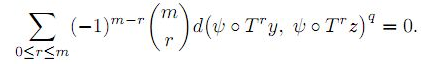2: Paper Source PDF document

Paper's Title:

Salagean-type Harmonic Univalent Functions with Respect to Symmetric Points

Author(s):

R. A. Al-Khal and H. A. Al-Kharsani

Department of Mathematics, Faculty of Science, Girls College,
P.O. Box 838,
Dammam, Saudi Arabia
ranaab@hotmail.com
hakh@hotmail.com

Abstract:

A necessary and sufficient coefficient are given for functions in a class of complex-valued harmonic univalent functions of the form f=h+\g using Salagean operator where h and g are analytic in the unit disk U = {z:|z|<1}. Furthermore, distortion theorems, extreme points, convolution condition, and convex combinations for this family of harmonic functions are obtained.

2: Paper Source PDF document

Paper's Title:

Multivalent Harmonic Mappings Convoluted With a Multivalent Analytic Function

Author(s):

Om P. Ahuja and Özlem Güney

Kent State University, Department of Mathematical Sciences,
14111, Claridon-Troy Road, Burton, Ohio 44021,
U.S.A.
oahuja@kent.edu

University of Dicle, Department of Mathematics,
Faculty of Science and Art, 21280 Diyarbakir,
Turkey
ozlemg@dicle.edu.tr

Abstract:

The object of this paper is to study certain geometric properties of a family of multivalent harmonic mappings in the plane convoluted with a multivalent analytic function in the open unit disc.

2: Paper Source PDF document

Paper's Title:

Generalizing Polyhedra to Infinite Dimension

Author(s):

Paolo d'Alessandro

Department of Mathematics, Third University of Rome,
Lgo S.L. Murialdo 1, 00146 Rome, Italy.

dalex@mat.uniroma3.it.

URL: http://www.mat.uniroma3.it/users/dalex/dalex.html.

Abstract:

This paper generalizes polyhedra to infinite dimensional Hilbert spaces as countable intersections of closed semispaces. Highlights are the structure theory that shows that a polyhedron is the sum of compact set (in a suitable topology) plus a closed pointed cone plus a closed subspace, giving the internal representation of polyhedra. In the final part the dual range space technique is extended to the solution of infinite dimensional LP problems.

2: Paper Source PDF document

Paper's Title:

Harmonic Functions with Positive Real Part

Author(s):

Sďbel Yalçin

Uludag Universitesi,
Fen Edebiyat Fakultesi, Matematik Bolumu,
16059 Bursa,
Turkey

syalcin@uludag.edu.tr

Abstract:

In this paper, the class of harmonic functions f=h+{g} with positive real part and normalized by f(ζ )=1, (|ζ|<1) is studied, where h and g are analytic in U={z:|z|<1}. Some properties of this class are searched. Sharp coefficient relations are given for functions in this class. On the other hand, the author make use of Alexander integral transforms of certain analytic functions (which are starlike with respect to f(ζ)) with a view to investigating the construction of sense preserving, univalent and close to convex harmonic functions.

1: Paper Source PDF document

Paper's Title:

Multivalued Equilibrium Problems with Trifunction

Author(s):

Etisalat College of Engineering, P.O. Box 980, Sharjah, United Arab Emirates
noor@ece.ac.ae

Abstract:

In this paper, we use the auxiliary principle technique to suggest some new classes of iterative algorithms for solving multivalued equilibrium problems with trifunction. The convergence of the proposed methods either requires partially relaxed strongly monotonicity or pseudomonotonicity. As special cases, we obtain a number of known and new results for solving various classes of equilibrium and variational inequality problems. Since multivalued equilibrium problems with trifunction include equilibrium, variational inequality and complementarity problems as specials cases, our results continue to hold for these problems.

1: Paper Source PDF document

Paper's Title:

Essential Random Fixed Point Set of Random Operators

Author(s):

Ismat Beg

Centre for Advanced Studies in Mathematics,
Lahore University of Management Sciences (LUMS),
54792-Lahore, PAKISTAN. ibeg@lums.edu.pk
URL: http://web.lums.edu.pk/~ibeg

Abstract:

We obtain necessary and sufficient conditions for the existence of essential random fixed point of a random operator defined on a compact metric space. The structure of the set of essential random fixed points is also studied.

1: Paper Source PDF document

Paper's Title:

Inequalities for the Čebyšev Functional of Two Functions of Selfadjoint Operators in Hilbert Spaces

Author(s):

S. S. Dragomir

School of Engineering and Science
Victoria University, PO 14428
Melbourne City MC, Victoria 8001,
Australia

sever.dragomir@vu.edu.au
URL
: http://www.staff.vu.edu.au/RGMIA/dragomir/

Abstract:

Some recent inequalities for the Čebyšev functional of two functions of selfadjoint linear operators in Hilbert spaces, under suitable assumptions for the involved functions and operators, are surveyed.

1: Paper Source PDF document

Paper's Title:

Shape Diagrams for 2D Compact Sets - Part I: Analytic Convex Sets.

Author(s):

S. Rivollier, J. Debayle and J.-C. Pinoli

Ecole Nationale Supérieure des Mines de Saint-Etienne,

CIS - LPMG, UMR CNRS 5148, 158 cours Fauriel,

42023 Saint-Etienne Cedex 2, France.

Abstract:

Shape diagrams are representations in the Euclidean plane introduced to study 3-dimensional and 2-dimensional compact convex sets. Such a set is represented by a point within a shape diagram whose coordinates are morphometrical functionals defined as normalized ratios of geometrical functionals. Classically, the geometrical functionals are the area, the perimeter, the radii of the inscribed and circumscribed circles, and the minimum and maximum Feret diameters. They allow thirty-one shape diagrams to be built. Most of these shape diagrams can also been applied to more general compact sets than compact convex sets. Starting from these six classical geometrical functionals, a detailed comparative study has been performed in order to analyze the representation relevance and discrimination power of these thirty-one shape diagrams. The purpose of this paper is to present the first part of this study, by focusing on analytic compact convex sets. A set will be called analytic if its boundary is piecewise defined by explicit functions in such a way that the six geometrical functionals can be straightforwardly calculated. The second and third part of the comparative study are published in two following papers [19.20]. They are focused on analytic simply connected sets and convexity discrimination for analytic and discretized simply connected sets, respectively.

1: Paper Source PDF document

Paper's Title:

Properties of Certain Multivalent Functions Involving Ruscheweyh Derivatives

Author(s):

N-Eng Xu and Ding-Gong Yang

Department of Mathematics,
Changshu Institute of Technology,
Changshu, Jiangsu 215500,
China

Abstract:

Let Ap(p∈ N) be the class of functions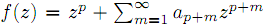which are analytic in the unit disk. By virtue of the Ruscheweyh derivatives we introduce the new subclasses Cp(n,α,β,λ,μ) of Ap. Subordination relations, inclusion relations, convolution properties and a sharp coefficient estimate are obtained. We also give a sufficient condition for a function to be in Cp(n,α,β,λ,μ)

1: Paper Source PDF document

Paper's Title:

On the Hyers-Ulam Stability of Homomorphisms and Lie Derivations

Author(s):

Department of Mathematics, Payame Noor University,
P.O. Box: 19395-3697, Tehran,
Iran.

Abstract:

Let A be a Lie Banach*-algebra. For each elements (a, b) and (c, d) in A2:= A * A, by definitions

(a, b) (c, d)= (ac, bd),
|(a, b)|= |a|+ |b|,
(a, b)*= (a*, b*),

A2 can be considered as a Banach*-algebra. This Banach*-algebra is called a Lie Banach*-algebra whenever it is equipped with the following definitions of Lie product: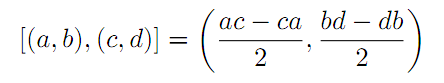for all a, b, c, d in A. Also, if A is a Lie Banach*-algebra, then D: A2→A2 satisfying

D ([ (a, b), (c, d)])= [ D (a, b), (c, d)]+ [(a, b), D (c, d)]

for all \$a, b, c, d∈A, is a Lie derivation on A2. Furthermore, if A is a Lie Banach*-algebra, then D is called a Lie* derivation on A2 whenever D is a Lie derivation with D (a, b)*= D (a*, b*) for all a, b∈A. In this paper, we investigate the Hyers-Ulam stability of Lie Banach*-algebra homomorphisms and Lie* derivations on the Banach*-algebra A2.

1: Paper Source PDF document

Paper's Title:

Presentation a mathematical model for bone metastases control by using tamoxifen

Author(s):

Maryam Nikbakht, Alireza Fakharzadeh Jahromi and Aghileh Heydari

Department of Mathematics,
Payame Noor University,
P.O.Box 19395-3697, Tehran,
Iran.
.E-mail: maryam_nikbakht@pnu.ac.ir

Department of Mathematics,
Faculty of Basic Science,
Shiraz University of Technology.

Department of Mathematics,
Payame Noor University,
P.O.Box 19395-3697, Tehran,
Iran.
E-mail: a-heidari@pnu.ac.ir

Abstract:

Bone is a common site for metastases (secondary tumor) because of breast and prostate cancer. According to our evaluations the mathematical aspect of the effect of drug in bone metastases has not been studied yet. Hence, this paper suggested a new mathematical model for bone metastases control by using tamoxifen. The proposed model is a system of nonlinear partial differential equations. In this paper our purpose is to present a control model for bone metastases. At end by some numerical simulations, the proposed model is examined by using physician.

1: Paper Source PDF document

Paper's Title:

Polynomial Dichotomy of C0-Quasi Semigroups in Banach Spaces

Author(s):

Sutrima1,2, Christiana Rini Indrati2, Lina Aryati2

1Department of Mathematics,
Universitas Sebelas Maret,
PO Box 57126, Surakarta,
Indonesia.
E-mail: sutrima@mipa.uns.ac.id

2Department of Mathematics,
PO Box 55281, Yogyakarta,
Indonesia.
E-mail: rinii@ugm.ac.id, lina@ugm.ac.id

Abstract:

Stability of solutions of the problems is an important aspect for application purposes. Since its introduction by Datko , the concept of exponential stability has been developed in various types of stability by various approaches. The existing conditions use evolution operator, evolution semigroup, and quasi semigroup approach for the non-autonomous problems and a semigroup approach for the autonomous cases. However, the polynomial stability based on C0-quasi semigroups has not been discussed in the references. In this paper we propose a new stability for C0-quasi semigroups on Banach spaces i.e the polynomial stability and polynomial dichotomy. As the results, the sufficient and necessary conditions for the polynomial and uniform polynomial stability are established as well as the sufficiency for the polynomial dichotomy. The results are also confirmed by the examples.

1: Paper Source PDF document

Paper's Title:

Euler-Maclaurin Formulas for Functions of Bounded Variation

Author(s):

G. De Marco, M. De Zotti, C. Mariconda

Dipartimento di Matematica Tullio Levi-Civita,
Italy.
E-mail:  carlo.mariconda@unipd.it
URL: http://www.math.unipd.it

Abstract:

The first-order Euler-Maclaurin formula relates the sum of the values of a smooth function on an interval of integers with its integral on the same interval on R. We formulate here the analogue for functions that are just of bounded variation.

1: Paper Source PDF document

Paper's Title:

Inequalities for Functions of Selfadjoint Operators on Hilbert Spaces:
a Survey of Recent Results

Author(s):

Sever S. Dragomir1,2

1Mathematics, College of Engineering & Science
Victoria University, PO Box 14428
Melbourne City, MC 8001,
Australia
E-mail: sever.dragomir@vu.edu.au

2DST-NRF Centre of Excellence in the Mathematical and Statistical Sciences,
School of Computer Science & Applied Mathematics,
University of the Witwatersrand,
Private Bag 3, Johannesburg 2050,
South Africa
URL: https://rgmia.org/dragomir

Abstract:

The main aim of this survey is to present recent results concerning inequalities for continuous functions of selfadjoint operators on complex Hilbert spaces. It is intended for use by both researchers in various fields of Linear Operator Theory and Mathematical Inequalities, domains which have grown exponentially in the last decade, as well as by postgraduate students and scientists applying inequalities in their specific areas.

1: Paper Source PDF document

Paper's Title:

The Higher Coefficients for Bazilevic Functions B1(α)

Author(s):

Department of Mathematics,
Faculty of Mathematics and Natural Sciences,,
Brawijaya University, Malang Jawa Timur 65145
Indonesia.
E-mail: marjono@ub.ac.id

Abstract:

Let f be analytic in D{z: |z|< 1} with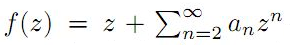, and normalized by the conditions f(0)=f'(0)-1=0. We give sharp estimates for the seventh and eighth coefficients for the class of Bazilevic functions with logarithmic growth, B1α, defined by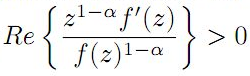for α≥0.

1: Paper Source PDF document

Paper's Title:

Coexisting Attractors and Bubbling Route to Chaos in Modified Coupled Duffing Oscillators

Author(s):

B. Deruni1, A. S. Hacinliyan1,2, E. Kandiran3, A. C. Keles2, S. Kaouache4, M.-S. Abdelouahab4, N.-E. Hamri4

1Department of Physics,
University of Yeditepe,
Turkey.

2Department of Information Systems and Technologies,
University of Yeditepe,
Turkey

3Department of Software Development,
University of Yeditepe,
Turkey.

4Laboratory of Mathematics and their interactions,
University Center of Abdelhafid Boussouf,
Mila 43000,
Algeria.

E-mail: berc890@gmail.com
ahacinliyan@yeditepe.edu.tr
engin.kandiran@yeditepe.edu.tr
cihan.keles@yeditepe.edu.tr
s.kaouache@centr-univ-mila.dz
medsalah3@yahoo.fr
n.hamri@centre-univ-mila.dz

Abstract:

In this article dynamical behavior of coupled Duffing oscillators is analyzed under a small modification. The oscillators have cubic damping instead of linear one. Although single duffing oscillator has complex dynamics, coupled duffing systems possess a much more complex structure. The dynamical behavior of the system is investigated both numerically and analytically. Numerical results indicate that the system has double scroll attractor with suitable parameter values. On the other hand, bifurcation diagrams illustrate rich behavior of the system, and it is seen that, system enters into chaos with different routes. Beside classical bifurcations, bubbling route to chaos is observed for suitable parameter settings. On the other hand, Multistability of the system is indicated with the coexisting attractors, such that under same parameter setting the system shows different periodic and chaotic attractors. Moreover, chaotic synchronization of coupled oscillators is illustrated in final section.

1: Paper Source PDF document

Paper's Title:

On Statistically Φ-Convergence

Author(s):

Supama

Department of Mathematics,
Yogyakarta 55281,
Indonesia.
E-mail: supama@ugm.ac.id

Abstract:

The idea of statistical convergence was introduced by Antoni Zygmund in 1935. Based on in the idea of Zygmund, Henry Fast and Hugo Steinhaus independently introduced a concept of statistical convergence as a generalization of an ordinary convergence in the same year 1951. In this paper, by using the Orlicz function, we introduce a concept of statistical Φ-convergence, as a generalization of the statistical convergence. Further, we observe some basic properties and some topological properties of the statistical Φ-convergent sequences

1: Paper Source PDF document

Paper's Title:

Fekete Szegö problem on the Class of Bazilevič functions B1(α) related to the Lemniscate Bernoulli

Author(s):

N. M. Asih, Marjono, Sa'adatul Fitri, Ratno Bagus Edy Wibowo

Department of Mathematics,
University of Brawijaya,
Malang 65145,
Indonesia.
Department of Mathematics,
University of Udayana,
Bali,
Indonesia.

Department of Mathematics,
University of Brawijaya,
Malang 65145,
Indonesia.
E-mail: marjono@ub.ac.id

Department of Mathematics,
University of Brawijaya,
Malang 65145,
Indonesia.

Department of Mathematics,
University of Brawijaya,
Malang 65145,
Indonesia.
E-mail: rbagus@ub.ac.id

Abstract:

We provide a sharp boundaries inequalities for Fekete Szegö problem |a3-μ a22|, the coefficients of logarithmic function log~ f(z)/z, and the coefficients of the inverse function f(f'(w)) on the Bazilevič functions B1(α) related to the Lemniscate Bernoulli on the unit disk D={z: |z| < 1}. We obtained the result by using some properties of function with positive real part relates to coefficients problems.

Search and serve lasted 0 second(s).